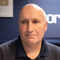Study Notes

# Decision Trees

Level:
AS, A-Level
Board:
AQA, Edexcel, OCR, IB

Last updated 22 Mar 2021

A decision tree is a mathematical model used to help managers make decisions.

• A decision tree uses estimates and probabilities to calculate likely outcomes.
• A decision tree helps to decide whether the net gain from a decision is worthwhile.

Let's look at an example of how a decision tree is constructed. We'll use the following data:

A decision tree starts with a decision to be made and the options that can be taken. Don't forget that there is always an option to decide to do nothing!

The first task is to add possible outcomes to the tree (note: circles represent uncertain outcomes)

Next we add in the associated costs, outcome probabilities and financial results for each outcome.

These probabilities are particularly important to the outcome of a decision tree.

Probability is

• The percentage chance or possibility that an event will occur
• Ranges between 1 (100%) and 0
• If all the outcomes of an event are considered, the total probability must add up to 1

Finally we complete the maths in the model by calculating:

Expected value:

The financial value of an outcome calculated by multiplying the estimated financial effect by its probability

Net gain:

The value to be gained from taking a decision.

Net gain is calculated by adding together the expected value of each outcome and deducting the costs associated with the decision.

Let's look at the calculations. What do they suggest is the best option?

Option: Launch loyalty card:

High sales: (0.6 x £1,000,000) = £600,000

Low sales: (0.4 x £750,000) = £300,000

Total expected value = £900,000

Net gain: £900,000 - £500,000 = £400,000

Option: Cut prices:

High sales: (0.8 x £800,000) = £640,000

Low sales: (0.2 x £500,000) = £100,000

Total expected value = £740,000

Net gain: £740,000 - £300,000 = £440,000

Both options indicate a positive net gain, suggesting that either would be better than doing nothing.

However, cutting prices has a slightly higher net gain & looks the best option of the two considered.

BENEFITS OF USING DECISION TREES

• Choices are set out in a logical way
• Potential options & choices are considered at the same time
• Use of probabilities enables the “risk” of the options to be addressed
• Likely costs are considered as well as potential benefits
• Easy to understand & tangible results

DRAWBACKS OF USING DECISION TREES

• Probabilities are just estimates – always prone to error
• Uses quantitative data only – ignores qualitative aspects of decisions
• Assignment of probabilities and expected values prone to bias
• Decision-making technique doesn’t necessarily reduce the amount of risk

### Scientific Decision Making

Topic Videos### Lunchtime Learning with the Calculation Practice Book!

2nd February 2018

### The dangers of short-term strategy

5th January 2018### Resource of the Week - AQA and Edexcel A Level Business

16th October 201725th August 2017

Topic Videos

### Uber and The Pros and Cons of Product ( or Service) Development

12th November 2016

### Save the baby or the painting?! A great starter on short-termism!

6th November 2016

© 2002-2022 Tutor2u Limited. Company Reg no: 04489574. VAT reg no 816865400.